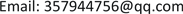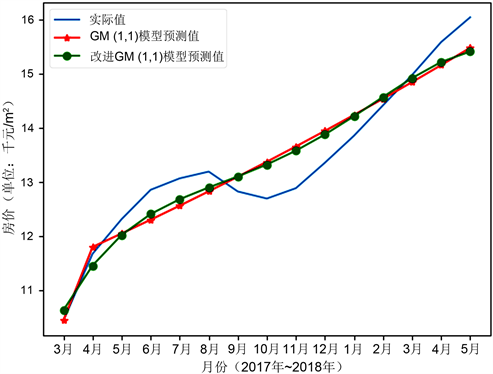﻿ 基于改进GM模型的房价预测模型 The Housing Price Forecasting Model Based on Improved GM Model

Computer Science and Application
Vol. 08  No. 12 ( 2018 ), Article ID: 27991 , 9 pages
10.12677/CSA.2018.812200

The Housing Price Forecasting Model Based on Improved GM Model

Haiqing Chen, Shuangyuan Wang, Zihao Li, Jun Xia, Jinming Wei

School of Computer Engineering, Qingdao University of Technology, Qingdao ShandongReceived: Nov. 28th, 2018; accepted: Dec. 6th, 2018; published: Dec. 13th, 2018ABSTRACT

In view of the dynamic changes characteristics of real estate price, this article takes the real estate price of The West Coast New Area of Qingdao as an example, combines the improved grey GM (1, 1) forecast model with Markov model, and puts forward a new time series basing forecast model to forecast the real estate price of The West Coast New Area of Qingdao. The grey GM (1, 1) model is suitable for short-term data forecasting with fewer data and fewer fluctuations, while the Markov model is suitable for prediction processes with large data fluctuations. By combining the advantages of the improved GM (1, 1) model with the Markov model, a new forecast model is established for real estate price forecasting. The experimental results show that the accuracy of this model is high, and it is a feasible model for house price forecasting.

Keywords:GM (1, 1) Model, Polynomial Fitting, Markov Model, Forecast, Real Estate PriceCopyright © 2018 by authors and Hans Publishers Inc.

This work is licensed under the Creative Commons Attribution International License (CC BY).

http://creativecommons.org/licenses/by/4.0/1. 引言

2. 灰色–马尔可夫模型基本原理

2.1. 灰色GM (1, 1)模型

2.1.1. GM (1, 1)模型基本形式

${X}^{\left(0\right)}=\left({X}^{{}^{\left(0\right)}}\left(1\right)\text{},{X}^{{}^{\left(0\right)}}\left(2\right)\text{},{X}^{{}^{\left(0\right)}}\left(3\right)\cdots {X}^{\left(0\right)}\left(n\right)\right)$

${X}^{\left(1\right)}=\left({X}^{\left(1\right)}\left(1\right)\text{},{X}^{\left(1\right)}\left(2\right)\text{},{X}^{{}^{\left(1\right)}}\left(3\right)\cdots {X}^{\left(1\right)}\left(n\right)\right)$

${X}^{\left(1\right)}\left(i\right)=\sum {}_{\left(k=1\right)}^{i}{X}^{\left(0\right)}\left(k\right),i=1,2,3,\cdots ,n$

${Z}^{\left(1\right)}=\left({Z}^{\left(1\right)}\left(2\right),{Z}^{\left(1\right)}\left(3\right)\cdots {Z}^{\left(1\right)}\left(n\right)\right)$

${Z}^{\left(1\right)}\left(k\right)=\frac{{X}^{\left(1\right)}\left(k\right)+{X}^{\left(1\right)}\left(k-1\right)}{2},k=2,3,\cdots ,n$

${X}^{\left(0\right)}\left(k\right)+\alpha \cdot {Z}^{\left(1\right)}\left(k\right)=b$

2.1.2. GM (1, 1)模型作用机理

$\frac{\text{d}{x}^{\left(1\right)}\left(k\right)}{\text{d}t}+\alpha {x}^{\left(1\right)}\left(k\right)=\mu$ (1)

$\frac{\text{d}{x}^{\left(1\right)}\left(k\right)}{\text{d}t}+\alpha {z}^{\left(1\right)}\left(k\right)=\text{μ}$

$\frac{\text{d}{x}^{\left(1\right)}\left(k\right)}{\text{d}t}=\frac{{X}^{\left(1\right)}\left(k\right)-{X}^{\left(1\right)}\left(k-1\right)}{1},k=2,3,\cdots ,n$

${X}^{\left(1\right)}\left(k\right)-{X}^{\left(1\right)}\left(k-1\right)+\alpha {z}^{\left(1\right)}\left(k\right)=\text{μ},k=2,3,\cdots ,n$ (2)

$\left[\begin{array}{c}\alpha \\ \text{μ}\end{array}\right]={\left({B}^{T}B\right)}^{-1}{B}^{T}Y$

$B=\left[\begin{array}{cc}-{z}^{\left(1\right)}\left(2\right)& 1\\ \begin{array}{c}-{z}^{\left(1\right)}\left(3\right)\\ ⋮\\ -{z}^{\left(1\right)}\left(n\right)\end{array}& \begin{array}{c}1\\ ⋮\\ 1\end{array}\end{array}\right]Y=\left[\begin{array}{c}{x}^{\left(0\right)}\left(2\right)\\ \begin{array}{c}{x}^{\left(0\right)}\left(3\right)\\ ⋮\end{array}\\ {x}^{\left(0\right)}\left(n\right)\end{array}\right]$

${\stackrel{^}{X}}^{\left(1\right)}\text{=}c{e}^{-\alpha t}+\frac{\text{μ}}{\alpha }$ (3)

${\stackrel{^}{X}}^{\left(1\right)}\left(k+1\right)=c{e}^{-\alpha k}+\frac{\text{μ}}{\alpha },k=0,1,2,3,\cdots n-1$ (4)

${\stackrel{^}{X}}^{\left(1\right)}\left(1\right)=c+\frac{\text{μ}}{\alpha }={X}^{\left(0\right)}\left(1\right)$

${\stackrel{^}{X}}^{\left(1\right)}\left(k+1\right)=\left({X}^{\left(0\right)}\left(1\right)-\frac{\text{μ}}{\alpha }\right){e}^{-\alpha k}+\frac{\text{μ}}{\alpha }k=0,1,2,3，\cdots ，n-1$ (5)

${\stackrel{^}{X}}^{\left(0\right)}\left(k+1\right)={\stackrel{^}{X}}^{\left(1\right)}\left(k+1\right)-{\stackrel{^}{X}}^{\left(1\right)}\left(k\right),k=1,2,3,\cdots ,n-1$ (6)

${\stackrel{^}{X}}^{\left(0\right)}\left(k+1\right)=\left({X}^{\left(0\right)}\left(1\right)-\frac{\text{μ}}{\alpha }\right){e}^{-\alpha k}\cdot \left(1-{e}^{\alpha }\right)$ (7)

2.2. 马尔可夫模型

$P\left\{{x}_{n+1}={i}_{n+1}|{x}_{0}={i}_{0},{x}_{1}={i}_{1},\cdots {x}_{n}={i}_{n}\right\}=P\left\{{x}_{n+1}={i}_{n+1}|{x}_{n}={i}_{n}\right\}$

2.2.1. 状态区域划分

2.2.2. 状态转移概率矩阵

${P}_{ij}^{\left(k\right)}=\frac{{m}_{ij}^{\left(k\right)}}{{M}_{i}}$

${m}_{ij}^{\left(k\right)}$ 表示状态Ei经过k步转移到状态Ej的次数，Mi为状态Ei出现的总次数。

${P}^{\left(k\right)}\text{=}|\begin{array}{cc}\begin{array}{cc}{P}_{11}^{\left(k\right)}& {P}_{12}^{\left(k\right)}\\ {P}_{21}^{\left(k\right)}& {P}_{22}^{\left(k\right)}\end{array}& \begin{array}{cc}\cdots & {P}_{1n}^{\left(k\right)}\\ \cdots & {P}_{2n}^{\left(k\right)}\end{array}\\ \begin{array}{cc}⋮& ⋮\\ {P}_{n1}^{\left(k\right)}& {P}_{n2}^{\left(k\right)}\end{array}& \begin{array}{cc}\dots & ⋮\\ \dots & {P}_{nn}^{\left(k\right)}\end{array}\end{array}|$

2.2.3. 计算预测值

3. 改进的灰色模型

1) 传统GM (1, 1)算法：

${X}^{\left(0\right)}\left(t+1\right)=\left(1-{e}^{-0.02092}\right)\cdot \left(10.437-\frac{11.4556}{-0.02092}\right){e}^{0.02092t}$

2) 利用二次多项式拟合改进：

$Y\left(x\right)=0.12538{X}^{2}+11.428837X-0.707217$

3) 利用三次多项式拟合改进：

$Y\left(x\right)=0.010056{X}^{3}-0.115960{X}^{2}+13.023698X-3.16889$

4) 利用四次多项式拟合改进：

$Y\left(x\right)=-0.000504{X}^{4}+0.0182631{X}^{3}-0.2263819{X}^{2}+1.383664X+9.4540461$

5) 利用指数多项式拟合改进：

$Y\left(x\right)=14.5871851128\cdot {e}^{-\frac{{}^{0.420828817852}}{x}}$

6) 利用对数多项式拟合改进：

$Y\left(x\right)=1.7297367+\mathrm{log}\left(x\right)+10.13768756$

4. 应用实例

4.1. 建立改进的GM (1, 1)模型Table 1. Comparison of GM (1, 1) model and modified GM (1, 1) model

X(0) = (10.437, 11.670, 12.311, 12.855, 13.067, 13.192, 12.824, 12.693, 12.885, 13.357, 13.856, 14.420, 14.981, 15.590, 16.049 )

GM (1, 1)模型与改进的GM (1, 1)模型，对原始实际数据的拟合效果如图1所示：Figure 1. Comparison of the fitting effect between GM (1, 1) model and modified GM (1, 1) model

4.2. 利用马尔可夫模型修正

4.2.1. 状态区间划分

4.2.2. 构建状态转移概率矩阵

${P}^{\left(1\right)}=\left[\begin{array}{cc}\begin{array}{cc}\frac{2}{3}& \frac{1}{3}\\ \frac{1}{4}& \frac{1}{4}\end{array}& \begin{array}{cc}0& 0\\ \frac{1}{4}& \frac{1}{4}\end{array}\\ \begin{array}{cc}0& 0\\ 0& \frac{1}{6}\end{array}& \begin{array}{cc}0& 1\\ 0& \frac{5}{6}\end{array}\end{array}\right]\text{}{P}^{\left(2\right)}=\left[\begin{array}{cc}\begin{array}{cc}\frac{1}{3}& \frac{2}{3}\\ \frac{1}{4}& 0\end{array}& \begin{array}{cc}0& 0\\ \frac{1}{4}& \frac{2}{4}\end{array}\\ \begin{array}{cc}0& 0\\ \frac{1}{5}& \frac{1}{5}\end{array}& \begin{array}{cc}0& 1\\ 0& \frac{3}{5}\end{array}\end{array}\right]\text{}{P}^{\left(3\right)}=\left[\begin{array}{cc}\begin{array}{cc}0& \frac{2}{3}\\ 0& 0\end{array}& \begin{array}{cc}\frac{1}{3}& 0\\ 0& 1\end{array}\\ \begin{array}{cc}0& 0\\ \frac{2}{5}& \frac{1}{5}\end{array}& \begin{array}{cc}0& 1\\ 0& \frac{2}{5}\end{array}\end{array}\right]$Table 3. Results of state transition probability

$\frac{15.439}{1-2.78%}=15.880$

5. 结束语

The Housing Price Forecasting Model Based on Improved GM Model[J]. 计算机科学与应用, 2018, 08(12): 1804-1812. https://doi.org/10.12677/CSA.2018.812200

1. 1. 杨楠, 邢力聪. 灰色马尔可夫模型在房价指数预测中的应用[J]. 统计与信息论坛, 2006, 21(5): 52-55.

2. 2. 遇华仁, 莫军, 李劲. 基于灰色马尔可夫模型的市场需求预测[J]. 商业研究, 2009(11): 43-45.

3. 3. 谷秀娟, 李超. 基于马尔可夫链的房价预测研究[J]. 消费经济, 2012, 28(5): 40-42.

4. 4. 谢乃明, 刘思峰. 离散GM(1, 1)模型与灰色预测模型建模机理[J]. 系统工程理论与实践, 2005(1): 93-99.

5. 5. 杨华龙, 刘金霞, 郑斌. 灰色预测GM(1, 1)模型的改进及应用[J]. 数学的实践与认识, 2011, 41(23): 39-46.

6. 6. 李振平, 桂预风. 基于灰关联神经网络和马尔可夫模型的股票价格预测[J]. 内蒙古师范大学学报, 2016, 45(3): 310-314.

7. 7. 黄嘉仪, 张小洪. 基于灰色–马尔可夫模型的厦门市房价预测[J]. 福建建筑, 2012(1): 104-106.

8. 8. 胡岸. 灰色马尔可夫链组合在预测股票价格上的应用[J]. 科学技术与工程, 2012, 12(1): 133-136.

9. 9. 田红霞. 基于两次改进的灰色–马尔可夫模型的太原房价预测[J]. 哈尔滨师范大学自然科学学报, 2015, 31(3): 4-7.

10. 10. 李瑶, 曹菡, 马晶. 基于改进的灰色模型的旅游需求预测研究[J]. 计算机科学, 2018, 45(1): 122-127.

11. 11. 刘呈玲, 方红远, 刘志辉. 改进的灰色预测模型在区域用水总量预测中的应用[J]. 华北水利水电大学学报, 2018, 39(2): 57-62.